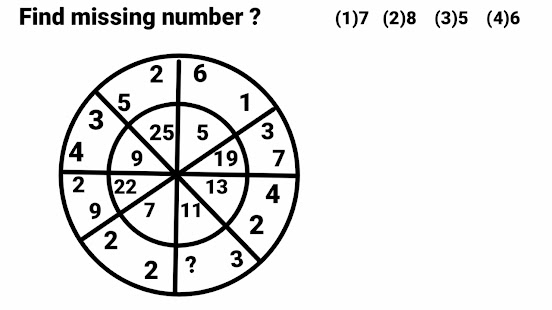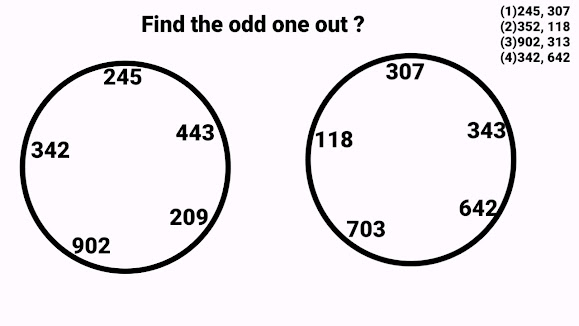## 10 Most Important Reasoning Problems of Circles with Solutions, How to Solve Reasoning Circle Problems

Ten Most  Important Reasoning problems of circles with solutions. These types of problems are very helpful for cracking competitive exams like ssc cgl, ssc chsl and various Bank exams and many other similar exams .

## Problem # 1

This circle consists of four quadrants and every  quadrant consists of three numbers , And every quadrant have two numbers in outer part and  1 number  is in the inner part . To find the value of question mark  "?"  , we shall use two numbers which are in the outer part to calculate the value of the number which is in the inner part of every quadrant .

Square of 8 × Taking Power 4 of  3  =  64 × 81  = 5184

Now add all these digits  5 + 1 + 8 + 4 = 18

Now reversing the order of these digits obtained in  previous step

18   <----> 81 The number in the inner part

Square of 5 ×  Taking Power 4 of  7  =  25 ×  2401  = 60025

Now add all these digits  6 + 0 + 0 + 2  + 5  = 13

Now reversing the order of these digits obtained in  previous step

13   <---->  31 The number in the inner part

Square of 8 × Taking Power 4 of  9  =  64 × 6561  = 419904

Now add all these digits  4 + 1 + 9 + 9 + 0 + 4 = 27

Now reversing the order of these digits obtained in  previous step

27   <----> 72 The number in the inner part

Square of 7 × Taking Power 4 of  7  =  49 × 2401 = 117649

Now add all these digits  1 + 1 + 7 + 6 + 4 + 9  = 28

Now reversing the order of these digits obtained in  previous step

28   <----> 82 The number in the inner part

Option (1) is correct option.

### Problem # 2This  circle has been divided into eight sectors. Every sector  consist of three numbers. To solve this problem multiply both the numbers  in any sector which are in the outer part and then add 1 to it ,the result so obtained is written in the inner part of the sector which is exactly opposite to this sector .Continuing in this manner  we shall have  all the numbers placed accordingly. .  Starting from the sector immediately to the right of question mark.

( 2 × 4 ) + 1  =  9  ( In the inner part of 5th sector )

( 3 × 7 ) + 1 =  22  ( In the inner part of 6th sector )

( 1 × 6 ) + 1 =  7  ( In the inner part of  7th sector )

( 5 × 2 ) + 1  = 11  ( In the inner part of 8th sector )

( 3 × 4 ) + 1  =  13  ( In the inner part of 1st sector )

( 2 × 9 ) + 1  =  19  ( In the inner part of 2nd sector )

( 2 × 2 ) + 1  =  5  ( In the inner part of 3rd sector )

( ? × 3 ) + 1  =  25  ( In the inner part of 4th sector )

Now we have to find the value of  "?" like this

( ? × 3 ) + 1  =  25

? × 3  =  25 - 1

? × 3  =  24

? = 24/3

? = 8

Hence option (2)  is correct option

### Problem # 3

Sq (3 + 4 ) + Sq (4 ) = Sq 7 + Sq 4 = 49 + 16 = 65 (The number is in circle opposite to 3 and 4)

Sq (7 + 2 ) + Sq (7 ) = Sq 9 + Sq 7 = 81 + 49 = 130 (The number is in circle opposite to 7 and 2)

Sq (6 + 9 ) + Sq (9 ) = Sq 15 + Sq 9 =  225 + 81 = 306  (The number is in circle opposite to 6 and 9)

Sq (5 + 8 ) + Sq (8 ) = Sq 13 + Sq 8 = 169 + 64 = 233 (The number is in circle opposite to 5 and 8)
Hence option (4) is right answer.

### Problem # 4All the Numbers except one in both the circles are written in same pattern . If we add all the three digits of any number in both the circles then we shall get same result except in one number in both the circles. And that different result will be the odd one out in this problem.

### In 1st circle

The sum of digits of number 319 = 3 + 1 +  9  = 13  ( Odd one out)
The sum of digits of number 441 = 4 + 4 + 1 = 9
The sum of digits of number 243 = 2 + 4 + 3 = 9
The sum of digits of number 612 =  6 + 1 + 2 = 9
The sum of digits of number 342 = 3 + 4 + 2 =  9

### In 2nd circle

The sum of digits of number 322 =  3 + 2 + 2 = 7
The sum of digits of number 313 =  3 + 1 + 3 = 7
The sum of digits of number 142 = 1 + 4 + 2 = 7
The sum of digits of number 304 = 3 + 0 + 4 = 7
The sum of digits of number 349 = 3+ 4 + 9 = 16 ( Odd one out)
So from both these circles two results 13 and 16 of numbers 319 and 349  are different from other.
Hence option (4) is right answer.

### Problem # 5

Since this circle consists of four quadrants and every quadrant consists of three numbers ,two numbers are in outer part and one number is in the inner part  ( triangle) . The  number which is in the inner part (triangle) can be found  using both the numbers which  are in the outer part of the same quadrant by using the following formula
(Difference of both  numbers  in the outer part) - ( difference of  both the digits in the triangular parts ) = 1

(12 - 8)  - ( 6- 3 ) = 4 - 3 = 1

(4 - 3)  - ( 1 - 1  ) = 1 - 0 = 1

(9 - 6)  - ( 6- 4 ) = 3 - 2 = 1

(12 - 10 )  - ( ? - ? ) = 2 - 1 = 1
If we put 43 in place of question mark only then difference of its digits  will be equal to 1 and in other cases the difference of digits is not equal to 1 which is necessarily required .
So the correct option will be  (1) .

### Problem # 6All the Numbers except one in both the circles are written in same pattern . If we add all the three digits of any number in both the circles then we shall get same result except in one number in both the circles. And that different result will be the odd one out in this problem.

In 1st circle

The sum of digits of number 245 = 2 + 4 + 5  = 11
The sum of digits of number 443 = 4 + 4 + 3 = 11
The sum of digits of number 209 = 2 + 0 + 9 = 11
The sum of digits of number 902 = 9 + 0 + 2 = 11
The sum of digits of number 342 = 3 + 4 + 2 =  9  ( Odd one out)

In 2nd circle

The sum of digits of number 307 =  3+ 0 + 7 = 10
The sum of digits of number 343 = 3 + 4 + 3 = 10
The sum of digits of number 642 = 6 + 4 + 2 = 12( Odd one out)
The sum of digits of number 703 = 7 + 0 + 3 = 10
The sum of digits of number 118 = 1 + 1 + 8 = 10
So from both these circles two results 9 and 12 of numbers 342 and 642  are different from other.
Hence option (4) is right answer.

# Problem # 7

3 - 1 = 2
6 - 3 = 3
11 - 6 = 5
18 - 11 = 7.
Since all these resultant numbers are prime numbers so next prime number should be 11
Therefore  ? - 18 = 11
This implies ? = 29.
Hence option (D) is correct option.

## Problem # 8

Here 1st circle consist of four parts and if we divide sum of all the digits in its outer part with number of parts then result will be the middle number.
( 6 + 2 + 9 + 7  )/4 =  24/4 = 6 ( Middle number).

2nd circle consist of five parts and if we divide sum of all the digits in its outer part with number of parts then result will be the middle number.
( 7 + 2 + 5 + 8 + 3 )/5 = 25/5 =5 ( Middle number).

3rd circle consist of six parts and if we divide sum of all the digits in its outer part with number of parts then result will be  the middle number.
(  8 + 6 + 7 + 5 + 7 + 9 )/6 = 42/6 =7 ( Middle number).
Hence option (3) is correct answer

## Problem # 9

Starting clockwise from the question mark i. e.  from 3 . These numbers 3, 5 ,7 ,11 ,13 are written in a pattern of prime number series. So so after 13  the next prime number will be 17. But 17 is not in the  given option. Hence this series will start from the prime number  prior to 3 . The number prior to 3 is 2 . Because smallest prime number is 2.
Hence pattern will be 2 , 3 , 5, 7 , 11, 13.
Therefore correct option will be (D) .
Also read these posts on Reasoning
******************************

## Problem # 10

This Circle consist of four quadrant and every quadrant consists of three numbers. Here the number in the outer part of every sector contribute to the number which is in the inner part of that sector . Starting from the sector which is below the question mark , out of these two numbers written in Outer part , the the number which is greater/maximum of these two is written in the inner part.

#### In 1st Sector

Max (14,17 ) = 17 , Here greater of the two numbers will be selected

#### In 2nd Sector

Max (13,18) = 18, Here greater of the two numbers will be selected

#### In 3rd Sector

Max (5 , 3 ) = 5, Here greater of the two numbers will be selected

#### In 4th Sector

Max ( 4, 8 ) = 8, Here greater of the two numbers will be selected

So option (2)  will be correct option

Share: